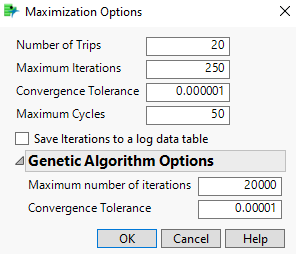Profilers > Profiler > Prediction Profiler Options > Maximization Options Window
Publication date: 11/10/2021

## Maximization Options Window

Figure 3.8 Maximization Options WindowNumber of Trips

The number of random starts in the optimization algorithm. Each trip starts with a randomly chosen value for each of the factors in the model.

Maximum Iterations

The maximum number of steps taken within each trip. If the algorithm does not converge before the maximum iterations is reached, a new trip is started.

Convergence Tolerance

The upper limit for the convergence criterion for the optimization algorithm. If the convergence criterion is less than this value for two consecutive iterations, the algorithm stops.

Maximum Cycles

(Not applicable if there are linear constraints.) The maximum number of cycles in the algorithm.

Genetic Algorithm Options

(Available only if there is a disallowed combination constraint or if one of the extrapolation control features is turned on.) Provides additional options for the genetic algorithm. The genetic algorithm is used only if the solution from the regular optimization algorithm is not feasible.

Maximum number of iterations

The maximum number of iterations for the genetic algorithm.

Convergence Tolerance

The upper limit for the convergence criterion for the genetic algorithm.

Caution: The solution found by the genetic algorithm is not guaranteed to be the global optimum. This is true for all genetic algorithms. A genetic algorithm is needed for optimization problems that have more general constraints. For example, JMP uses the genetic algorithm when a disallowed combinations constraint is present because these types of constraints can involve categorical factors, be nonlinear, or be any general constraint entered via JSL.